# Divisibility Rule : Download Mathematics Study Notes Free PDF For Teaching ExamMathematics is an equally important section for UPTET, MPTET, KVS & DSSSB Exams and has even more abundant importance in some other exams conducted by central or state govt. Generally, there are questions asked related to basic concepts, Facts of the Divisibility Rule.

To let you make the most of Mathematics section, we are providing important facts related to the Divisibility Rule. At least 2-3 questions are asked from this topic in most of the teaching exams. We wish you all the best of luck to come over the fear of the Mathematics section.

How to Overcome Exam Fever, Especially When You Fear Maths

## Divisibility Rule

Divisibility by 2 If Last digit of the number is divisible by 2

Ex.: 92, 76, 112 are divisible by 2

Divisibility of 3 All such numbers the Sum of whose digits are divisible by 3

Ex.: When 335 is added to 5A7, the result is 8B2 is divisible by 3. What is the largest possible value of A?

Sol.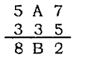A → 1, 2, 3, 4, 5 &

B → 5, 6, 7, 8, 9

8B2 is exactly ∴ 8 + B + 2 = multiple of 3

∴ B = 5 or 8 ⟹ A = 1 or 4

Divisibility by 4 If Last two digits of the number are divisible by 4

Ex.: Take the number 6316. Consider the last two digits 16. As 16 is divisible by 4, the original number 6316 is also divisible by 4.

Divisibility by 5 If Last digit (0 and 5) is divisible by 5

Ex.: 100, 195, 118975 are divisible by 5

Divisibility by 6 A number is divisible by 6 If it is simultaneously divisible by 2 and 3

Ex.: 834, the number is divisible by 2 as the last digit is 4.

The sum of digits is 8+3+4 = 15, which is also divisible by 3.

Hence 834 is divisible by 6.

Practice More Mathematics  Quiz Here

Divisibility by 7 Double the last digit and subtract it from the remaining leading truncated number. If the result is divisible by 7, then so was the original number.

Ex.: Check to see if 203 is divisible by 7

Sol.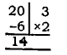Step I. Double the last digit = 3 × 2

= 6

Step.2 Subtract that from the rest of the Number = 20 – 6 = 14

Step.3 Check to see if the difference is divisible by 7. 14 is divisible by 7 therefore 203 is also divisible by 7

Divisibility by 8 If Last three digits of the number are divisible by 8

Divisibility of 9 All such numbers the Sum of whose digits are divisible by 9

Ex.: If 5432*7 is divisible by 9, then the digit in place of * is

Sol.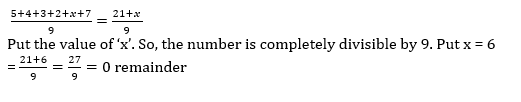Divisibility by 11 The difference of the sum of the digits in the odd places and the sum of digits in the even places is ‘O’ or multiple of 11 is divisible

How To Score 25+ Marks In Mathematics For CTET Exam?

Ex.: If * is a digit such that 5824* is divisible by 11, then * equals:

Sol: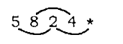⟹ 5 + 2 + * = 8 + 4

7 + * = 12

* = 12 – 7 = 5

Divisibility by 16 If Last four digits of the number are divisible by 16

Divisibility by 25 If Last two digits of the number are divisible by 25

Divisibility by 32 If Last five digits of the number are divisible by 32

Divisibility by 125 If Last three digits of the number are divisible by 125

Divisibility by 3, 7, 11, 13, 21, 37 and 1001 (i) If any number is made by repeating a digit 6 times the number will be divisible by 3, 7, 11, 13, 21, 37 and 1001 etc.

(ii) A six digit number if formed by repeating a three digit number; for example, 256, 256 or 678, 678 etc. Any number of this form is always exactly divisible by 7, 11, 13, 1001 etc.

Some important points

(a) If a is divisible by b then ac is also divisible by b.

(b) If a is divisible by b and b is divisible by c then a is divisible by c.

(c) If n is divisible by d and m is divisible by d then (m + n) and (m-n) are both divisible by d. This has an important implication. Suppose 48 and 528 are both divisible by 8.  Then (528 + 48) as well as (528 – 48) are divisible by 8)

Mathematics Study Notes For All Teaching Exams

Successive Division : If the quotient in a division is further used as a dividend for the next divisor and again the latest obtained divisor is used as a dividend for another divisor and so on, then it is called ” successive division” i.e, if we divide 150 by 4, we get 37 as quotient and 2 as a remainder then if 37 it divided by another divisor say 5 then we get 7 as a quotient and 2 remainder and again if we divide 7 by another divisor say 3 we get 2 as quotient and 1 as a remainder i.e, we can represent it as following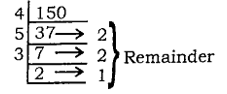Now you can see that the quotient obtained in the first division behaves as a dividend for another divisor 5. Once again the quotient 7 is treated as a dividend for the next divisor 3. Thus it is clear from the above discussion as

 Dividend Divisor Quotient Reminder 150 37 7 4 5 3 37 7 2 2 2 1

So, the 150 is successively divided by 4, 5, and 3 the corresponding remainders are 2, 2 and 1.

Sharing is caring!

×

Thank You, Your details have been submitted we will get back to you.Join India's largest learning destination

What You Will get ?

•Job Alerts
•Daily Quizzes
•Subject-Wise Quizzes
•Current Affairs
•Previous year question papers
•Doubt Solving session

ORJoin India's largest learning destination

What You Will get ?

•Job Alerts
•Daily Quizzes
•Subject-Wise Quizzes
•Current Affairs
•Previous year question papers
•Doubt Solving session

ORJoin India's largest learning destination

What You Will get ?

•Job Alerts
•Daily Quizzes
•Subject-Wise Quizzes
•Current Affairs
•Previous year question papers
•Doubt Solving session

Enter the email address associated with your account, and we'll email you an OTP to verify it's you.Join India's largest learning destination

What You Will get ?

•Job Alerts
•Daily Quizzes
•Subject-Wise Quizzes
•Current Affairs
•Previous year question papers
•Doubt Solving session

Enter OTP

Please enter the OTP sent to
/6

Did not recive OTP?

Resend in 60sJoin India's largest learning destination

What You Will get ?

•Job Alerts
•Daily Quizzes
•Subject-Wise Quizzes
•Current Affairs
•Previous year question papers
•Doubt Solving sessionJoin India's largest learning destination

What You Will get ?

•Job Alerts
•Daily Quizzes
•Subject-Wise Quizzes
•Current Affairs
•Previous year question papers
•Doubt Solving session

Almost there

+91Join India's largest learning destination

What You Will get ?

•Job Alerts
•Daily Quizzes
•Subject-Wise Quizzes
•Current Affairs
•Previous year question papers
•Doubt Solving session

Enter OTP

Please enter the OTP sent to Edit Number

Did not recive OTP?

Resend 60

By skipping this step you will not recieve any free content avalaible on adda247, also you will miss onto notification and job alerts

Are you sure you want to skip this step?

By skipping this step you will not recieve any free content avalaible on adda247, also you will miss onto notification and job alerts

Are you sure you want to skip this step?